# 论文原文¶

https://arxiv.org/abs/1612.01105

# 摘要¶

$\quad$本文提出的金字塔池化模块( pyramid pooling module)能够聚合不同区域的上下文信息,从而提高获取全局信息的能力。实验表明这样的先验表示(即指代PSP这个结构)是有效的，在多个数据集上展现了优良的效果。

# 介绍¶

$\quad$场景解析(Scene Parsing)的难度与场景的标签密切相关。先大多数先进的场景解析框架大多数基于FCN，但FCN存在的几个问题： - Mismatched Relationship：上下文关系匹配对理解复杂场景很重要，例如在上图第一行，在水面上的大很可能是“boat”，而不是“car”。虽然“boat和“car”很像。FCN缺乏依据上下文推断的能力。 - Confusion Categories： 许多标签之间存在关联，可以通过标签之间的关系弥补。上图第二行，把摩天大厦的一部分识别为建筑物，这应该只是其中一个，而不是二者。这可以通过类别之间的关系弥补。 - Inconspicuous Classes：模型可能会忽略小的东西，而大的东西可能会超过FCN接收范围，从而导致不连续的预测。如上图第三行，枕头与被子材质一致，被识别成到一起了。为了提高不显眼东西的分割效果，应该注重小面积物体。 $\quad$总的来说，FCN不能有效的处理场景之间的信息和全局信息，为了对FCN的这些缺点加以克服，提出了PSPNet。可以融合合适的全局特征，将局部和全局信息融合到一起，并提出了一个适度监督损失的优化策略，在多个数据集state of art。 $\quad$论文的主要贡献为: - 提出了一个金字塔场景解析网络，能够将难解析的场景信息特征嵌入基于FCN预测框架中 - 在基于深度监督损失ResNet上制定有效的优化策略 - 构建了一个实用的系统，用于场景解析和语义分割，并包含了实施细节

# 相关工作¶

$\quad$受到深度神经网络的驱动，场景解析和语义分割获得了极大的进展。例如FCN，ENet等工作。许多深度卷积神经网络为了扩大高层feature的感受野，常用空洞卷积，coarse-to-fine结构等方法。本文基于先前的工作，使用了带dilated卷积的FCN。 $\quad$大多数语义分割模型的工作基于2个方面： - 具有多尺度的特征融合，高层特征具有强的语义信息，底层特征包含更多的细节。 - 基于结构预测。例如使用CRF(条件随机场)做后端细化分割结果。 $\quad$为了充分的利用全局特征层次先验知识来进行不同场景理解，本文提出的PSP模块能够聚合不同区域的上下文从而达到获取全局上下文的目的。

# PSP网络¶

## 3.2 PSP 模块¶

$\quad$在CNN中感受野可以粗略的被认为是使用上下文信息的大小，论文指出在许多网络中没有充分的获取全局信息，所以效果不好。要解决这一问题，常用方法是： - 用全局平均池化处理：但这在某些数据上上可能会失去空间关系导致模糊。 - 由金字塔池化产生不同层次的特征最后被平滑的连接层一个FC层做分类。这样可以去除CNN固定大小的图像分类约束，减少不同区域之间的信息损失。 论文提出了一个具有层次全局优先级，包含不同子区域之间的不同尺度信息，称之为pyramid pooling module。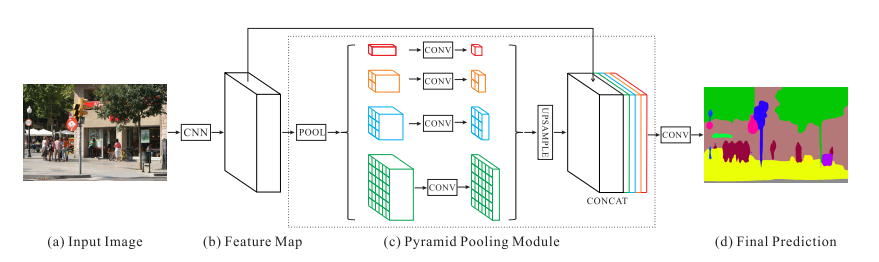$\quad$该模块融合了4种不同金字塔尺度的特征，第一行红色是最粗糙的特征–全局池化生成单个bin输出，后面三行是不同尺度的池化特征。为了保证全局特征的权重，如果金字塔共有N个级别，则在每个级别后使用1×11×1的卷积将对于级别通道降为原本的1/N。再通过双线性插值获得未池化前的大小，最终concat到一起。 $\quad$金字塔等级的池化核大小是可以设定的，这与送到金字塔的输入有关，论文中使用的4个等级，核大小分别为$1\times1$,$2\times2$,$3\times3$,$6\times6$。还需要注意一点的是，基础层经过预训练的模型(ResNet101)和空洞卷积策略提取feature map,提取后的feature map是输入的⅛大小。feature map经过Pyramid Pooling Module得到融合的带有整体信息的feature，再上采样并和池化前的feature map相concat。最后过一个卷积层得到最终输出。PSPNet本身提供了一个全局上下文的先验(即指代Pyramid Pooling Module这个结构)，后面的实验会验证这一结构的有效性。

# 基于ResNet的深度监督网络¶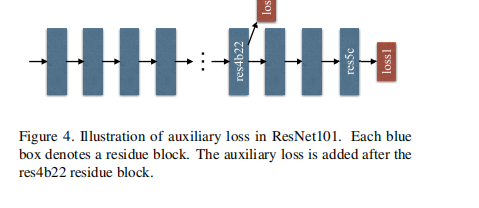PSPNet的基础网络层是在ResNet101的基础上做了改进，除了使用后面的softmax分类做loss，额外的在第四阶段添加了一个辅助的loss，两个loss一起传播，使用不同的权重，共同优化参数。后续的实验证明这样做有利于快速收敛。

# 实验¶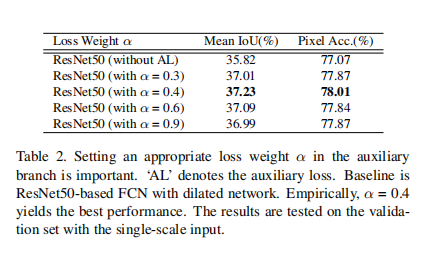实验都是以ResNet50-Baseline为基准，最后以α=0.4α=0.4为最佳。 - 测试预训练模型的深度：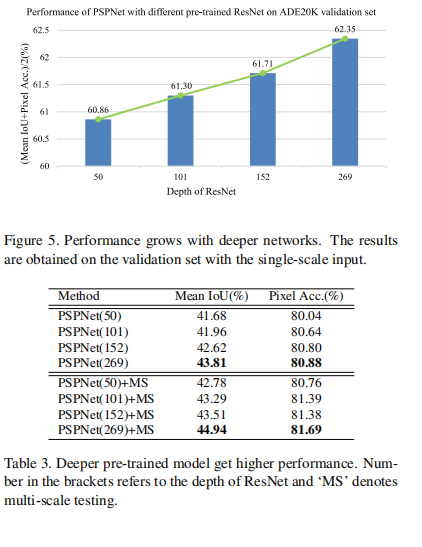可以看到在测试的{50,101,152,269}这四个层次的网络中，网络越深，效果越好。 - 多种技巧融合： -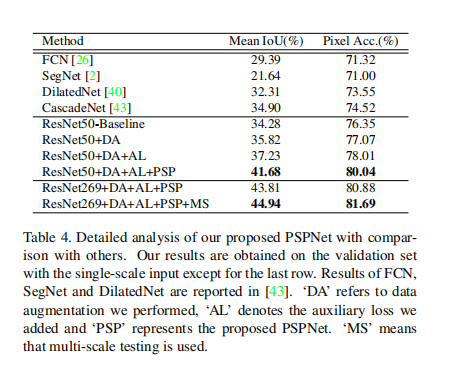ImageNet上的表现：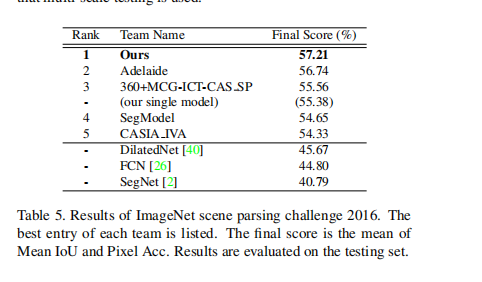PascalVOC上的表现：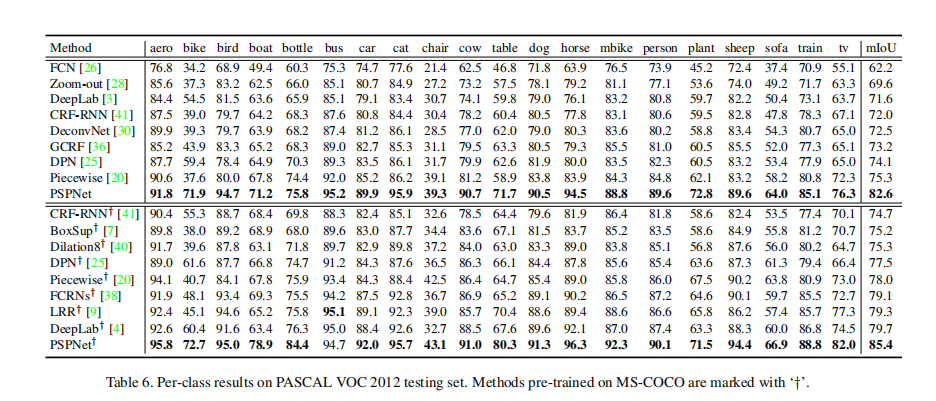Cityscapes上的表现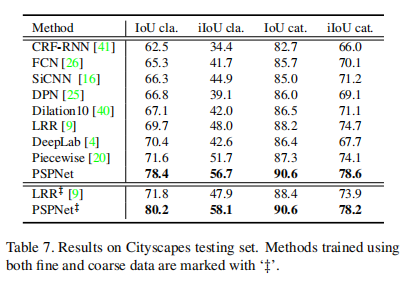# 代码实现¶

#coding=utf-8
from keras.models import *
from keras.layers import *
import keras.backend as K

def resize_image(inp, s):
return Lambda(lambda  x: K.resize_images(x, height_factor=s, width_factor=s, data_format='channels_last',
interpolation='bilinear'))(inp)

def pool_block(inp, pool_factor):
h = K.int_shape(inp)
w = K.int_shape(inp)
pool_size = strides = [int(np.round( float(h) / pool_factor)), int(np.round( float(w )/ pool_factor))]
x = Conv2D(512, (1, 1), padding='same', use_bias=False)(x)
x = BatchNormalization()(x)
x = Activation('relu')(x)
#print(strides)
x = resize_image(x, strides)
return x

def PSPNet(nClasses, optimizer=None, input_width=384, input_height=576):

assert input_height%192 == 0
assert input_width%192 == 0

input_size = (input_height, input_width, 3)
inputs = Input(input_size)
kernel = 3
filter_size = 64
pool_size = 2
x = inputs
x = (Conv2D(filter_size, (kernel, kernel), padding='valid'))(x)
x = (BatchNormalization())(x)
x = (Activation('relu'))(x)
x = (MaxPooling2D((pool_size, pool_size)))(x)
f1 = x

x = (Conv2D(128, (kernel, kernel), padding='valid'))(x)
x = (BatchNormalization())(x)
x = (Activation('relu'))(x)
x = (MaxPooling2D((pool_size, pool_size)))(x)
f2 = x

x = (Conv2D(256, (kernel, kernel), padding='valid'))(x)
x = (BatchNormalization())(x)
x = (Activation('relu'))(x)
x = (MaxPooling2D((pool_size, pool_size)))(x)
f3 = x

# x = (Conv2D(256, (kernel, kernel), padding='valid'))(x)
# x = (BatchNormalization())(x)
# x = (Activation('relu'))(x)
# x = (MaxPooling2D((pool_size, pool_size)))(x)
# f4 = x

# x = (Conv2D(256, (kernel, kernel), padding='valid'))(x)
# x = (BatchNormalization())(x)
# x = (Activation('relu'))(x)
# x = (MaxPooling2D((pool_size, pool_size)))(x)
# f5 = x

o = f3
pool_factors = [1, 2, 3, 6]
pool_outs = [o]
for p in pool_factors:
pooled = pool_block(o, p)
pool_outs.append(pooled)
o = Concatenate(axis=3)(pool_outs)
o = Conv2D(512, (1, 1), use_bias=False)(o)
o = BatchNormalization()(o)
o = Activation('relu')(o)

o = Conv2D(nClasses, (3, 3), padding='same')(o)
o = resize_image(o, (8, 8))
o_shape = Model(inputs, o).output_shape
outputHeight = o_shape
outputWidth = o_shape

o = Reshape((nClasses, input_height * input_width))(o)
o = Permute((2, 1))(o)
model = Model(inputs, o)
model.outputWidth = outputWidth
model.outputHeight = outputHeight

return model